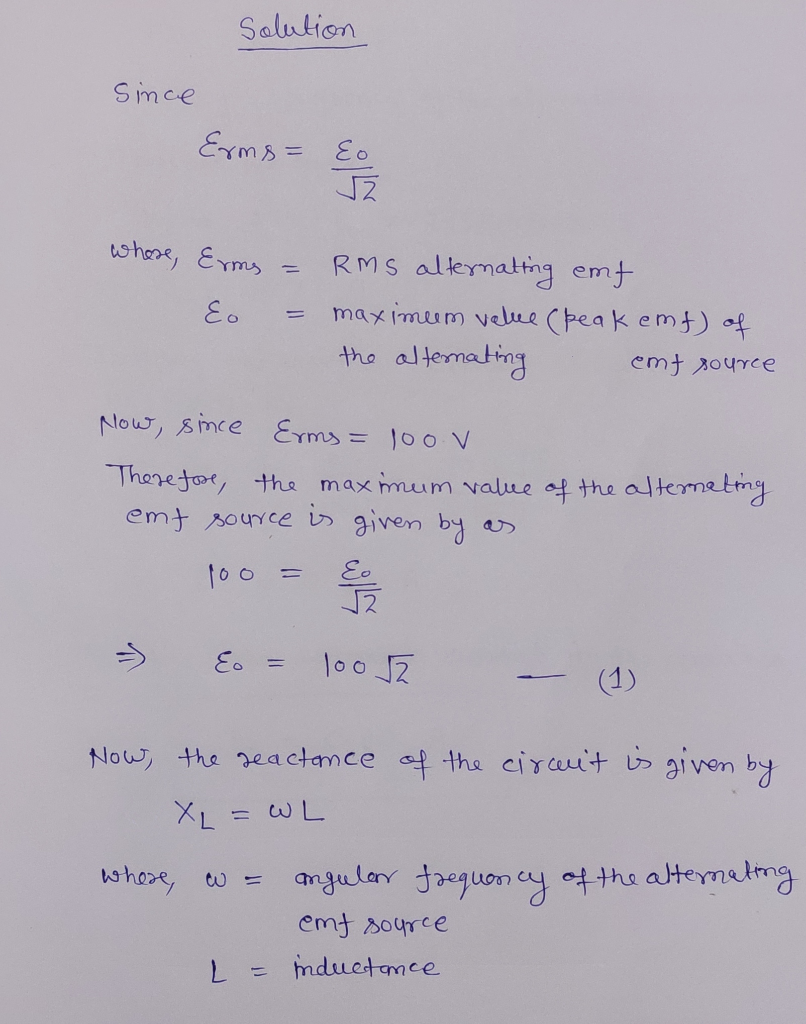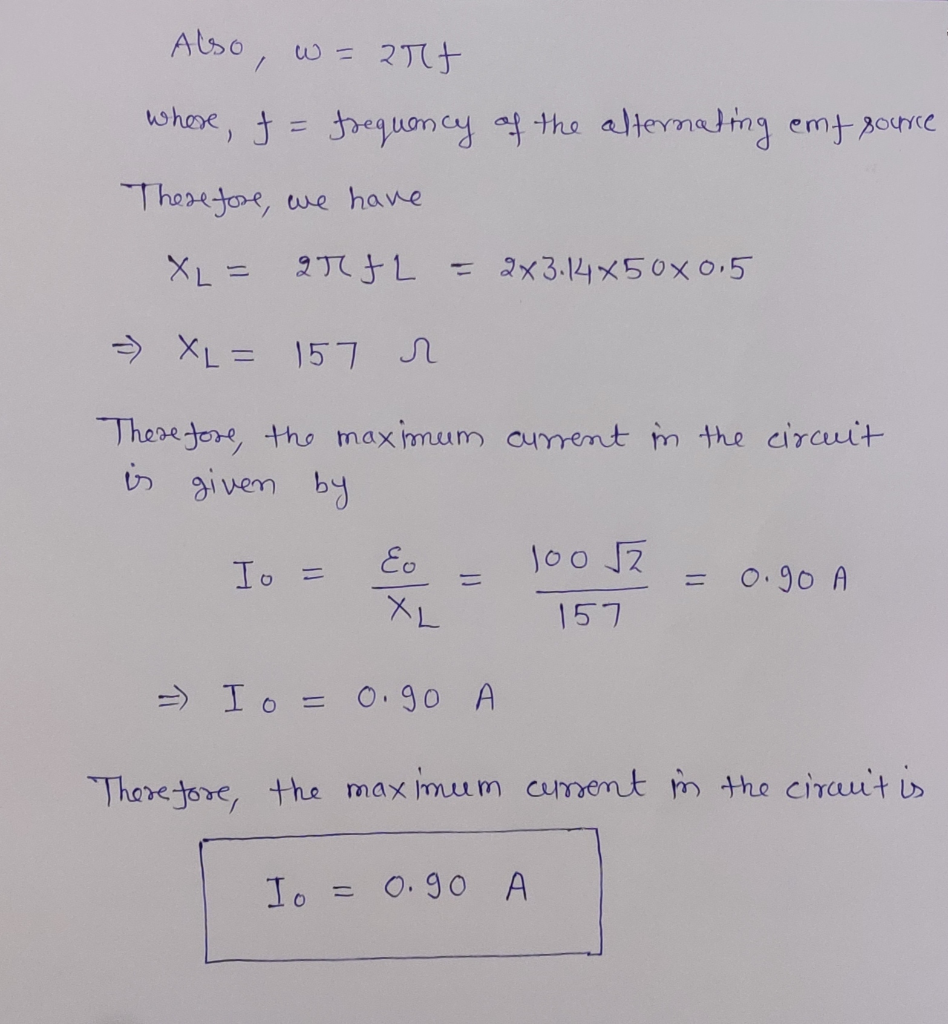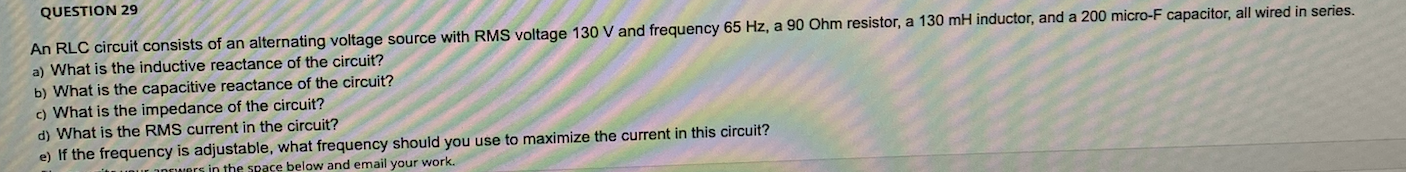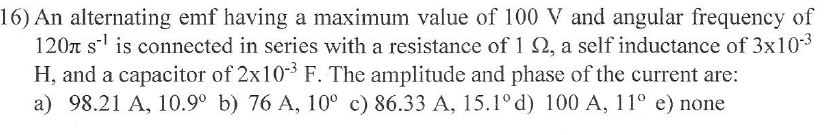Question

A 100 V RMS alternating emf source with frequency 50 Hz is connected to a 0.5 H inductor. What is the maximum current in the circuit?

 a. 0.6 A b. 5.65 A c. 90 A d. 0.14 A#### Earn Coins

Coins can be redeemed for fabulous gifts.

Similar Homework Help Questions
• ### An RLC circuit consists of an alternating voltage source with RMS voltage 90 V and frequency 100 Hz

An RLC circuit consists of an alternating voltage source with RMS voltage 90 V and frequency 100 Hz, a 180 Ohm resistor a 200 ml inductor, and a 900 micro-F capacitor, all wired in series. a) What is the inductive reactance of the circuit? b) What is the capacitive reactance of the circuit? c) What is the Impedance of the circuit? d) What is the RMS current in the circuit? e) of the frequency is adjustable, what frequency should you use to maximize the current...

• ### An RLC circuit consists of an alternating voltage source with RMS voltage 90 V and frequency...

An RLC circuit consists of an alternating voltage source with RMS voltage 90 V and frequency 100 Hz, 180 ohm resistor, 200mH inductor, and a 900 micro-F capacitor, all wired in series. A) What is inductive reactance of the circuit? B) What is the capacitive reactance of the circuit?C) What is the impedance  of the circuit? D)What is RMS current in the circuit?E) If the frequency is adjustable what frequency should you use to maximize the current in the circuit?

• ### QUESTION 29 An RLC circuit consists of an alternating voltage source with RMS voltage 130 V...QUESTION 29 An RLC circuit consists of an alternating voltage source with RMS voltage 130 V and frequency 65 Hz, a 90 Ohm resistor, a 130 mH inductor, and a 200 micro-F capacitor, all wired in series. a) What is the inductive reactance of the circuit? b) What is the capacitive reactance of the circuit? c) What is the impedance of the circuit? d) What is the RMS current in the circuit? e) If the frequency is adjustable, what frequency...

• ### An AC source has an output rms voltage of 71.0 V at a frequency of 41.5...

An AC source has an output rms voltage of 71.0 V at a frequency of 41.5 Hz. The source is connected across a 25.5-mH inductor. (a) Find the inductive reactance of the circuit. (b) Find the rms current in the circuit. (c) Find the maximum current in the circuit.

• ### Alternating source with amplitude ε = 12 V and frequency ωd = 100 rad/s is connected...

Alternating source with amplitude ε = 12 V and frequency ωd = 100 rad/s is connected in series with resistor R=300 Ω, capacitor C=25 μF and inductance L=2.0 H. What is the amplitude of the current in the circuit?

• ### A 45-mH inductor is connected to an ac source of emf with a frequency of 250...

A 45-mH inductor is connected to an ac source of emf with a frequency of 250 Hz and a maximum emf of 20 V. If the voltage across the inductor is +20 V at time t = 0, what is the voltage at time t = 2.0 ms? A : 0 V       B : −10 V       C :20 V     D : −20 V     E : 10 V

• ### An AC generator supplies an rms voltage of 120 V at 60.0 Hz. It is connected...

An AC generator supplies an rms voltage of 120 V at 60.0 Hz. It is connected in series with a 0.350 H inductor, a 5.10 μF capacitor and a 306 Ω resistor. a. What is the impedance of the circuit? b. What is the rms current through the resistor? c. What is the average power dissipated in the circuit? d. What is the peak current through the resistor? e. What is the peak voltage across the inductor? f. What is...

• ### An AC generator supplies an rms voltage of 120 V at 60.0 Hz. It is connected...

An AC generator supplies an rms voltage of 120 V at 60.0 Hz. It is connected in series with a 0.400 H inductor, a 5.30 ?F capacitor and a 251 ohm resistor. A.) What is the impedance of the circuit? B.) What is the rms current through the resistor? C.) What is the average power dissipated in the circuit? D.) What is the peak current through the resistor? E.) What is the peak voltage across the inductor F.) What is...

• ### A series LRC circuit is driven by an alternating source with rms voltage 700 V and...

A series LRC circuit is driven by an alternating source with rms voltage 700 V and angular frequency 500 rad/s. The circuit elements are a 400 Ω resistor, a 4.0 μF capacitor and a 0.40 H inductor. If the resistor and inductor are side by side, what would be the rms voltage across the two elements?

• ### 16) An alternating emf having a maximum value of 100 V and angular frequency of 1201...16) An alternating emf having a maximum value of 100 V and angular frequency of 1201 -' is connected in series with a resistance of 1 12, a self inductance of 3x10-3 H, and a capacitor of 2x10- F. The amplitude and phase of the current are: a) 98.21 A, 10.9° b) 76 A, 10° c) 86.33 A, 15.1°d) 100 A, 11° e) none